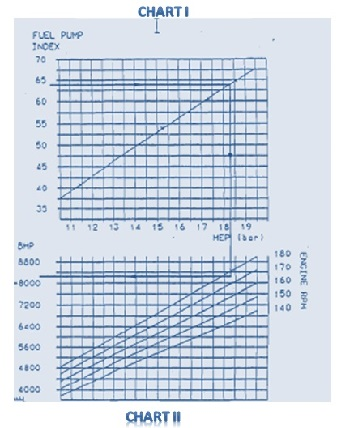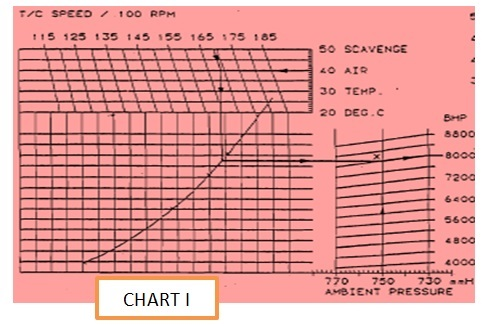MEO FUNCTION IV

# What is fuel pump index method and turbocharger speed method to calculate power of an engine?

1. ###### Fuel Pump Index method to calculate power
• The fuel pump index is used to find out the mean effective pressure from the nomogram graph. Again form the graph, the MEP at a specific speed gives the engine bhp.
• This method should only be used as a quick (rough) estimation, because the fuel oil, as well as the condition of the fuel pump, may have great effect on the index. In particular, worn fuel pumps or suction valves tend to increase the index, and will thus result in a too high power estimation.
• Chart I: Draw a horizontal line from the observed fuel pump index to the nomogram curve, and then a vertical line down to the observed engine speed on Chart II. From this intersection a horizontal line is drawn to the effective engine power scale.FIG.: FUEL PUMP INDEX METHOD

1. ###### Turbocharger speed method to calculate power

This method is more accurate than the Fuel pump index method. Chart I: draw a horizontal line from the observed scavenge air temp (Tscav) value and an inclined line from the observed turbocharger speed. From the intersection point, draw a vertical line down to the nomogram curve and then a horizontal line to the vertical line from the observed ambient pressure (point x in the ambient pressure scale). Finally, a line is drawn parallel with the inclined ‘ambient pressure correction’ lines. The effective engine power can then be read on the scale at the right hand side, i.e. 8,000 bhp.FIG: TURBOCHARGER SPEED METHOD